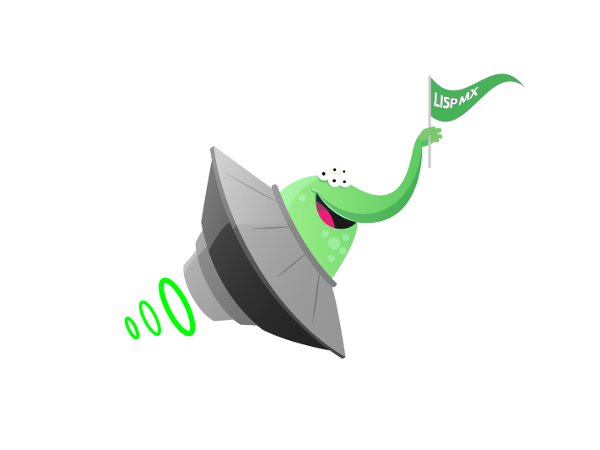lispEn 2018-02-14 18:18:58
365

# Prime number

Some days ago I ask me 'How can I create function that tell me if is a prime number or not, today I have created a simple function, so I would like to share it.'

I think that there are a lot of functions with the same result but I like doing code to know how it works.

(defun prime-number-p (number)

"Returns true if the number is prime; otherwise returns false"

(let ((counter 2)); the second prime number

(cond

;; special case

;; the definition of prime number

((equal number 1)

nil)

;; is a natural number which has exactly two distinct natural number divisors:

;;1 and itself.

;; apply the  prime number definition

(t

(loop

(when  (equal counter number)

(return t))

(if (= (mod number counter) 0)

(return nil))

(incf counter))))))

You can view the code :